## Chapter 06: An overview of scientific libaries¶

Further to Sympy, this lab sheet will give a brief overview of a number of popular Python libraries that can be used to tackle a number of different problems:

• numpy efficient numeric computations (for example for efficient linear algebra);
• matplotlib plotting;
• scipy a general purpose scientif library (for example include algorithms for numeric optimisation);
• networkx network/graph theory (for example to compute the chromatic number of a graph);
• pandas data manipulation (for example to read data from a given source and manipulate it);
• scikit-learn machine learning (this is one of Python's most popular libraries).

This lab sheet will not good a detailed overview of each library but aim to give a brief introduction to each.

### Numpy - http://www.numpy.org/¶

A video describing the library.

This is a fundamental library for efficient numeric computation. The building block is the numpy.array which can also be used to carry out linear algebraic manipulation.

Let us import numpy and define two 3 by 3 matrices:

In :
import numpy as np
A = np.array([[5, 1, -1], [-1, 2, 4], [1, 1, 1]])
B = np.array([[1, 2, 0], [-4, 2, 2], [1, 3, 1]])


We can access $A_{02}$ for example:

In :
A[0, 2]

Out:
-1

Or an entire row of $A$:

In :
A

Out:
array([ 5,  1, -1])

Or an entire column:

In :
A[:,1]

Out:
array([1, 2, 1])

We can do scalar multiplication:

In :
5 * A

Out:
array([[25,  5, -5],
[-5, 10, 20],
[ 5,  5,  5]])

We can raise the matrix to a high power:

In :
np.linalg.matrix_power(A, 20)

Out:
array([[33560473923573, 14496923401020,  6487017577736],
[ 1241032333047,   561674209891,   281855912501],
[ 9250938156331,  4004952911642,  1802706542938]])

We can carry out matrix addition:

In :
A + B

Out:
array([[ 6,  3, -1],
[-5,  4,  6],
[ 2,  4,  2]])

But also more complex things like matrix multiplication:

In :
np.dot(A, B)

Out:
array([[ 0,  9,  1],
[-5, 14,  8],
[-2,  7,  3]])

Recent versions of Python have @ as shorthand for matrix multiplication:

In :
A @ B

Out:
array([[ 0,  9,  1],
[-5, 14,  8],
[-2,  7,  3]])

We can also get the inverse and the determinant of a $A$:

In :
np.linalg.inv(A), np.linalg.det(A)

Out:
(array([[ 1. ,  1. , -3. ],
[-2.5, -3. ,  9.5],
[ 1.5,  2. , -5.5]]), -2.0)

Numpy also has numerous helpful functions that can be useful even if we are not doing any linear algebra. For example let us get an array of 100 values between -2 and 1:

In :
np.linspace(-2, 1, 10 ** 2)

Out:
array([-2.        , -1.96969697, -1.93939394, -1.90909091, -1.87878788,
-1.84848485, -1.81818182, -1.78787879, -1.75757576, -1.72727273,
-1.6969697 , -1.66666667, -1.63636364, -1.60606061, -1.57575758,
-1.54545455, -1.51515152, -1.48484848, -1.45454545, -1.42424242,
-1.39393939, -1.36363636, -1.33333333, -1.3030303 , -1.27272727,
-1.24242424, -1.21212121, -1.18181818, -1.15151515, -1.12121212,
-1.09090909, -1.06060606, -1.03030303, -1.        , -0.96969697,
-0.93939394, -0.90909091, -0.87878788, -0.84848485, -0.81818182,
-0.78787879, -0.75757576, -0.72727273, -0.6969697 , -0.66666667,
-0.63636364, -0.60606061, -0.57575758, -0.54545455, -0.51515152,
-0.48484848, -0.45454545, -0.42424242, -0.39393939, -0.36363636,
-0.33333333, -0.3030303 , -0.27272727, -0.24242424, -0.21212121,
-0.18181818, -0.15151515, -0.12121212, -0.09090909, -0.06060606,
-0.03030303,  0.        ,  0.03030303,  0.06060606,  0.09090909,
0.12121212,  0.15151515,  0.18181818,  0.21212121,  0.24242424,
0.27272727,  0.3030303 ,  0.33333333,  0.36363636,  0.39393939,
0.42424242,  0.45454545,  0.48484848,  0.51515152,  0.54545455,
0.57575758,  0.60606061,  0.63636364,  0.66666667,  0.6969697 ,
0.72727273,  0.75757576,  0.78787879,  0.81818182,  0.84848485,
0.87878788,  0.90909091,  0.93939394,  0.96969697,  1.        ])

### Matplotlib - https://matplotlib.org/¶

A video describing the library.

This is the most popular Python library for creating plots. We will illustrate first by creating a plot of the function:

$$-x ^ 4 + 9x ^ 2 + 4 x - 12$$

We do this by creating a set of $x$ values and computing the corresponding set of $f(x)$ values:

In :
def f(x):
return - x ** 4 + 9 * x ** 2 + 4 * x - 12

xs = np.linspace(-4, 5, 10 ** 3)  # Create 1000 points
ys = [f(x) for x in xs]


We are now ready to import and use matplotlib:

In :
import matplotlib.pyplot as plt

%matplotlib inline

plt.figure()
plt.plot(xs, ys)
plt.xlabel("$x$")
plt.ylabel("$y$")
plt.show()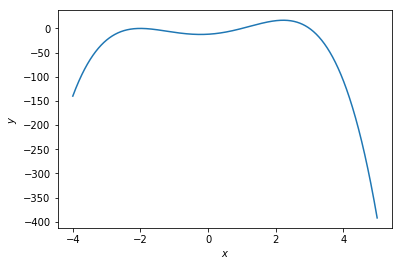Note the %matplotlib inline command which is a special Jupyter command to ensure plots are displayed in this notebook.

We can also save that image to a file that can be used elsewhere:

In :
plt.figure()
plt.plot(xs, ys)
plt.xlabel("$x$")
plt.ylabel("$y$")
plt.savefig("plot.pdf")  # experiment with .png, .jpg etc...Note that there are numerous other types of plots that can be obtained, for example here is a histogram of 1000 random values generated by numpy:

In :
np.random.seed(0)  # Setting a seed
values = np.random.normal(size=10 ** 3)
plt.figure()
plt.hist(values, bins=20)
plt.xlabel("$Value$")
plt.ylabel("Frequency")
plt.show()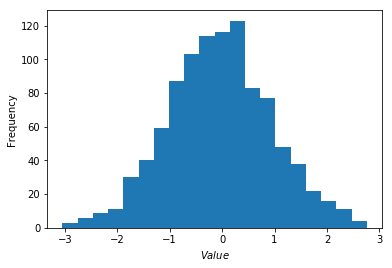### Scipy - https://docs.scipy.org/doc/scipy/reference/¶

A video describing the library.

Scipy is a library bringing together many different capabilities. One thing it can do is find roots using numeric approximation techniques:

In :
from scipy import optimize

In :
optimize.root(f, x0=0)

Out:
    fjac: array([[-1.]])
fun: array([0.])
message: 'The solution converged.'
nfev: 6
qtf: array([4.84234874e-12])
r: array([49.99999584])
status: 1
success: True
x: array([3.])
In :
f(3)

Out:
0

It can also minimize functions (of more than one variable):

In :
def g(x):
"""Here we assume g is a function of 2 variables and x is a vector"""
return np.cos(x) / (1 + x)

In :
optimize.minimize(g, x0=[0, 0])

Out:
      fun: 0.002697614111595088
hess_inv: array([[7.11535655e+06, 5.36889474e+00],
[5.36889474e+00, 1.00000405e+00]])
jac: array([-7.27712177e-06, -7.03148544e-07])
message: 'Optimization terminated successfully.'
nfev: 84
nit: 20
njev: 21
status: 0
success: True
x: array([3.6969793e+02, 2.6064249e-04])

We can also fit a function to data, here we will create some data that fits a curve, add some noise and try and recover the function:

In :
def func(x, a, b):
return a * x ** 2 + b

xs = np.linspace(0, 10, 50)
a_value, b_value = 1 / 2, 70
np.random.seed(0)
ys = [func(x, a=a_value, b=b_value) + 2 * np.random.random() for x in xs]


Let us take a look at the data:

In :
plt.figure()
plt.scatter(xs, ys)
plt.show()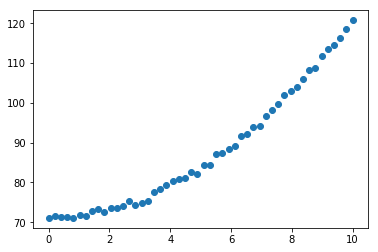In :
popt, pcov = optimize.curve_fit(func, xs, ys)


We recover the original used values of a and b:

In :
popt

Out:
array([ 0.49563406, 71.22294657])
In :
fitted_ys = [func(x, *popt) for x in xs]
plt.scatter(xs, ys, label="Original data")
plt.plot(xs, fitted_ys, label="Fitted data", color="red")
plt.show()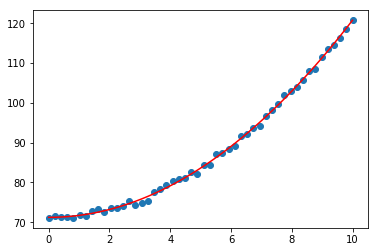### Networkx - https://networkx.github.io/¶

A video describing the library.

Networkx is used to handle graph theoretic objects. We can create a matrix in a number of ways, one of the simplest is by passing a set of edges. Here we import the library and create a graph object:

In :
import networkx as nx
edges = [(0, 1), (0, 2), (0, 3), (1, 2), (1, 3)]
G = nx.Graph(edges)
G

Out:
<networkx.classes.graph.Graph at 0x7ff96c1c1f28>

It is possible to obtain the adjacency matrix of the graph:

In :
M = nx.to_numpy_array(G)
M

Out:
array([[0., 1., 1., 1.],
[1., 0., 1., 1.],
[1., 1., 0., 0.],
[1., 1., 0., 0.]])

It is also possible to draw the graph:

In :
plt.figure()
nx.draw(G)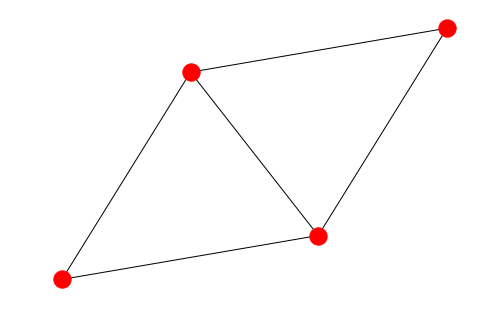We can also compute a graph coloring:

In :
coloring = nx.greedy_color(G)
coloring

Out:
{0: 0, 1: 1, 2: 2, 3: 2}

We see that the chromatic number of this graph is 3:

In :
len(set(coloring.values()))

Out:
3

### Pandas - https://pandas.pydata.org/¶

A video describing the library.

Pandas is Python's main library for data manipulation. As an example let us consider this dataset about extra marrital afairs: affairs.csv (download). This is data from the following research paper:

Fair, Ray C. "A theory of extramarital affairs." Journal of Political Economy 86.1 (1978): 45-61.

A pdf can be found here: https://fairmodel.econ.yale.edu/rayfair/pdf/1978A200.PDF

Let us import pandas and read the dataset in:

In :
import pandas as pd


We can look at the top of the data set:

In :
df.head()

Out:
sex age ym child religious education occupation rate nbaffairs
0 male 37.0 10.00 no 3 18 7 4 0
1 female 27.0 4.00 no 4 14 6 4 0
2 female 32.0 15.00 yes 1 12 1 4 0
3 male 57.0 15.00 yes 5 18 6 5 0
4 male 22.0 0.75 no 2 17 6 3 0

From the paper we read that the variables are:

• sex: The reported gender of the individual;
• age: The age of the individual;
• ym: Number of years married;
• child: Whether or not the individual has a child;
• religious: How religious the individual is (5: "very", 1: "not");
• education: Level of education (9: grade school, 20: PhD or MD);
• occupation: Occupation based on a scalle called the "Hollingshead classification";
• rate: Individual rating of marriage (5: "very happy", 1: "very unhappy").

We can obtain a quick overview of the data using the describe() method:

In :
df.describe()

Out:
age ym religious education occupation rate nbaffairs
count 601.000000 601.000000 601.000000 601.000000 601.000000 601.000000 601.000000
mean 32.487521 8.177696 3.116473 16.166389 4.194676 3.931780 1.455907
std 9.288762 5.571303 1.167509 2.402555 1.819443 1.103179 3.298758
min 17.500000 0.125000 1.000000 9.000000 1.000000 1.000000 0.000000
25% 27.000000 4.000000 2.000000 14.000000 3.000000 3.000000 0.000000
50% 32.000000 7.000000 3.000000 16.000000 5.000000 4.000000 0.000000
75% 37.000000 15.000000 4.000000 18.000000 6.000000 5.000000 0.000000
max 57.000000 15.000000 5.000000 20.000000 7.000000 5.000000 12.000000

We can also choose to slice our data set in specific ways, for example how is the mean number of affairs related to the rating of the marriage and whether or not the individual is male or female?

In :
df.groupby(["sex", "rate"])["nbaffairs"].mean()

Out:
sex     rate
female  1       4.545455
2       3.371429
3       1.782609
4       0.967742
5       0.823077
male    1       2.400000
2       4.548387
3       1.085106
4       1.623762
5       0.588235
Name: nbaffairs, dtype: float64

We can also choose to slice our data manually, for example let us only look at the data set for males:

In :
df[df["sex"] == "male"]

Out:
sex age ym child religious education occupation rate nbaffairs
0 male 37.0 10.000 no 3 18 7 4 0
3 male 57.0 15.000 yes 5 18 6 5 0
4 male 22.0 0.750 no 2 17 6 3 0
7 male 57.0 15.000 yes 2 14 4 4 0
9 male 22.0 1.500 no 4 14 4 5 0
10 male 37.0 15.000 yes 2 20 7 2 0
11 male 27.0 4.000 yes 4 18 6 4 0
12 male 47.0 15.000 yes 5 17 6 4 0
24 male 37.0 4.000 yes 2 20 6 4 0
27 male 42.0 15.000 yes 5 20 6 4 0
28 male 27.0 4.000 yes 3 16 5 5 0
30 male 42.0 15.000 yes 4 20 6 3 0
32 male 27.0 0.417 no 4 17 6 4 0
34 male 32.0 4.000 yes 1 18 6 4 0
38 male 22.0 1.500 yes 1 14 3 5 0
40 male 32.0 10.000 yes 5 20 6 5 0
41 male 52.0 15.000 yes 5 18 6 3 0
45 male 22.0 4.000 no 3 16 5 5 0
48 male 27.0 1.500 yes 4 16 3 5 0
49 male 42.0 15.000 yes 2 20 6 4 0
51 male 32.0 7.000 yes 2 20 6 4 0
52 male 27.0 4.000 yes 5 20 6 5 0
53 male 27.0 10.000 yes 4 20 6 4 0
54 male 22.0 4.000 no 1 18 5 5 0
56 male 22.0 1.500 yes 5 16 4 4 0
59 male 32.0 10.000 yes 4 20 6 4 0
61 male 37.0 10.000 yes 3 20 6 4 0
63 male 27.0 4.000 no 2 18 4 5 0
64 male 32.0 7.000 no 4 20 6 4 0
65 male 42.0 15.000 yes 2 17 3 5 0
... ... ... ... ... ... ... ... ... ...
548 male 42.0 15.000 yes 3 20 5 4 7
549 male 32.0 10.000 no 2 18 4 2 12
551 male 57.0 15.000 yes 5 20 4 5 7
552 male 47.0 15.000 yes 4 20 6 4 12
554 male 37.0 15.000 yes 3 17 6 3 12
555 male 37.0 15.000 yes 5 17 5 2 12
556 male 27.0 10.000 yes 2 20 6 4 7
557 male 37.0 15.000 yes 2 16 5 4 2
559 male 32.0 10.000 yes 3 17 6 3 7
560 male 37.0 15.000 yes 4 18 5 1 2
563 male 37.0 15.000 yes 2 17 5 4 12
567 male 52.0 7.000 no 4 16 5 5 12
574 male 32.0 4.000 yes 4 18 6 4 2
577 male 32.0 7.000 yes 4 16 4 4 1
578 male 37.0 15.000 no 3 14 6 2 12
579 male 42.0 15.000 yes 3 16 6 3 1
580 male 27.0 4.000 yes 1 18 5 4 1
581 male 37.0 15.000 yes 4 20 7 3 2
582 male 37.0 15.000 yes 3 20 6 4 7
583 male 22.0 1.500 no 2 12 3 3 3
584 male 32.0 4.000 yes 3 20 6 2 3
585 male 32.0 15.000 yes 5 20 6 5 2
587 male 47.0 15.000 no 1 18 6 5 12
591 male 42.0 15.000 yes 3 18 6 2 12
593 male 27.0 7.000 yes 2 17 5 4 12
594 male 32.0 10.000 yes 4 14 4 3 3
595 male 47.0 15.000 yes 3 16 4 2 7
596 male 22.0 1.500 yes 1 12 2 5 1
598 male 32.0 10.000 yes 2 17 6 5 2
599 male 22.0 7.000 yes 3 18 6 2 2

286 rows × 9 columns

We can combine this with matplotlib to get a plot of the mean number of affairs based on rating by gender:

In :
plt.figure()
for sex in ["male", "female"]:
plt.scatter(range(1, 5 + 1), df[df["sex"] == sex].groupby("rate")["nbaffairs"].mean(), label=sex)
plt.legend()
plt.xlabel("rate")
plt.ylabel("mean nbaffairs")
plt.show()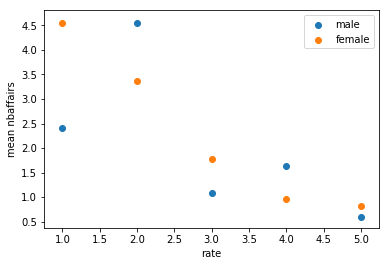### Scikit-learn - http://scikit-learn.org¶

A video describing the library.

The scikit learn library is a popular library for machine learning. As an example, let us aim to train a model that will predict whether or not someone will have an affair using the data above.

First let us create a new variable male and also change the child variable to be a boolean and store this data in a new dataframe called X which will be used to predict a new variable a new variable cheat (stored in y):

In :
df["bool_child"] = df["child"] == "yes"
df["male"] = df["sex"] == "male"
X = df[["age", "ym", "religious", "education", "rate", "occupation", "bool_child", "male"]]
y = df["nbaffairs"] > 0


Now let us import the specifit classifier for the algorithm we want to use:

In :
from sklearn.ensemble import RandomForestClassifier

/home/vince/anaconda3/envs/cfm/lib/python3.6/site-packages/sklearn/ensemble/weight_boosting.py:29: DeprecationWarning: numpy.core.umath_tests is an internal NumPy module and should not be imported. It will be removed in a future NumPy release.
from numpy.core.umath_tests import inner1d

In :
seed = 3
clf = RandomForestClassifier(random_state=seed)
clf.fit(X, y)

Out:
RandomForestClassifier(bootstrap=True, class_weight=None, criterion='gini',
max_depth=None, max_features='auto', max_leaf_nodes=None,
min_impurity_decrease=0.0, min_impurity_split=None,
min_samples_leaf=1, min_samples_split=2,
min_weight_fraction_leaf=0.0, n_estimators=10, n_jobs=1,
oob_score=False, random_state=3, verbose=0, warm_start=False)
In :
for feature, importance in zip(X.columns, clf.feature_importances_):
print(feature, round(importance, 5))

age 0.18605
ym 0.12635
religious 0.18442
education 0.16467
rate 0.13675
occupation 0.12495
bool_child 0.02848
male 0.04834


The most important feature seems to be the age of the individual. Let us use our trained model to predict whether or not some given individual is likely to have an affair over their lifetime:

In :
ages = range(35, 100)
probability_of_affair = []
for age in ages:
ym = age - 24
vince_knight = [[age, ym, 1, 20, 5, 3, True, True]]
probability_of_affair.append(clf.predict_proba(vince_knight))

plt.figure()
plt.plot(ages, probability_of_affair)
plt.xlabel("Vince's age")
plt.ylabel("Vince's probability of having an affair")
plt.ylim(0, 1)
plt.show()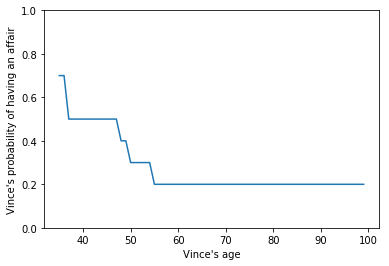#### Further resources for scikit-learn¶

This is a brief overview of the type of thing each library can do, depending on what you want to do be sure to explore them fully and there are many other libraries in the Python ecosystem.

Previous

Next

Source code: @drvinceknight Powered by: Python Jupyter Mathjax Github pages Skeleton css